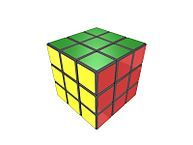Want to share your content on R-bloggers? click here if you have a blog, or here if you don't.Exercise 1
Create an array (3 dimensional) of 24 elements using the  `dim()`  function.

Exercise 2
Create an array (3 dimensional) of 24 elements using the  `array()`  function.

Exercise 3
Assign some dimnames of your choice to the array using the  `dimnames()`  function.

Exercise 4
Assign some dimnames of your choice to the array using the arguments of the  `array()`  function.

Exercise 5
Instead of column-major array, make a row-major array (transpose).

Exercise 6
For this exercise, and all that follow, download this file, and read it into R using the  `read.csv()`  function, e.g.:
```temp Copy the column named  N```  into a new variable  `arr`.

Exercise 7
Set dimensions of this variable and convert it into a 3 * 2 * 4 array. Add dimnames.

Exercise 8
Print the whole array on the screen.

Exercise 9
Print only elements of height 2, assuming the first dimension represents rows, the second columns and the third heigth.

Exercise 10
Print elements of height 1 and columns 3 and 1.

Exercise 11
Print element of height 2, column 4 and row 2.

Exercise 12
Repeat the exercises 9-11, but instead of using numbers to reference row, column and height, use  `dimnames`.

Image: Cubo completato” by Masakazu “Matto” Matsumoto from Nagoya, Japan – http://flickr.com/photos/vitroids/1527092739/. Licensed under CC BY 2.0 via Wikimedia Commons.